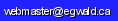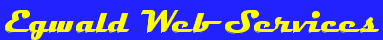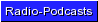Egwald Mathematics: Nonlinear Dynamics:

One Dimensional Dynamics and Bifurcations

by

Elmer G. Wiens

Egwald's popular web pages are provided without cost to users.
Please show your support by joining Egwald Web Services as a Facebook Fan:Follow Elmer Wiens on Twitter:One Dimensional Dynamics: Discrete Time Models.

Introduction

Continuous time, dynamic process are modelled with differential equations.  Discrete time, dynamic processes are modelled with difference equations. Consider a dynamic process where the rate of change of a variable from one time period to the next is proportional to magnitude of the variable.

Writing xt as the magnitude of the variable at time t, the dynamic process modelled as a difference equation is:

xt+1 = xt + k * xt = (1 + k) * xt,   x0 = x0,         (1)

where the constant k is the rate of change, and x0 is the magnitude of the variable at the start, time t = 0.

The solution to this difference equation is the discrete function of time, x(t, x0), for t an integer:

x(t, x0) = {x0, x1, x2,  . . .   xn,   ...   },

providing the time profile of the magnitude of x with an initial value of x0.

The sequence of numbers, {x0, x1, x2,  . . .   xn,   ...   }, is called an orbit.

One can solve the difference equation, (1), by induction:

 x1 = (1+ k) * x0, x2 = (1 + k) * x1 = (1 + k) * ( (1 + k) * x0) =(1 + k)2 * x0, x3 = (1 + k) * x2 = (1 + k) * ( (1 + k)2 * x0) = (1 + k)3 * x0,   . . .       . . .       . . . xt = (1 + k)t * x0   . . .       . . .       . . .

Writing λ = (1 + k), the difference equation (2) and its solution (3) are:

 xt+1 = λ * xt,   x0 = x0,         (2) x(t, x0) = λt * x0, for t an integer.         (3)

The solution x(t, x0) = λt * x0 suggests that the variable x will grow or decay exponentially from its starting population, x0, at a rate depending on the value of the parameter λ. If x0 > 0, and if λ > 1 (k > 0), the variable x increases in value; but if x0 > 0, and if λ < 1 (k < 0), the variable x decreases in value. Conversely, if x0 < 0, and if λ > 1 (k > 0), the variable x decreases in value; but if x0 < 0, and if λ < 1 (k < 0), the variable x increases in value.

One can see the evolution of these solutions in the following staircase diagrams:

In the first set of diagrams, the green lines touch the x-axis at x0, x1, x2, x3, etc. In both diagrams |x0| > |x1| > |x2| > |x3| > ... |xt| > ... → 0, but in the second diagram the xt alternate in sign.

 Orbit Converges:   -1 < λ < 1 Monotonic Convergence:   0 < λ < 1 Alternating Convergence:   -1 < λ < 0 y   =   x     versus     y   =   λ * x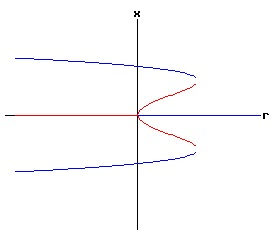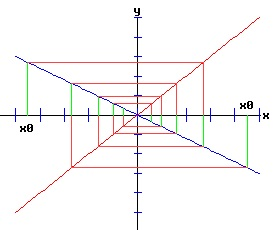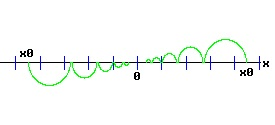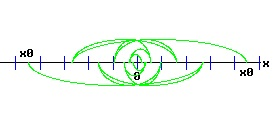The second set of diagrams above display the phase portraits of the difference equation, showing the movement of xt along the x-axis for t = 0, 1, 2, ... etc. This sequence converges to x* = 0 for any initial x0. The basin of attraction of x* = 0 is said to be the interval (-∞, ∞), since any initial x0 converges to 0.

In the first set of diagrams below, the green lines touch the x-axis at x0, x1, x2, x3, etc. In both diagrams 0 < |x0| < |x1| < |x2| < |x3| < ... < |xt| < ... → ∞ , but in the second diagram the xt alternate in sign.

 Orbit Diverges:   1 < |λ| Monotonic Divergence:   1 < λ Alternating Divergence:   λ < -1 y   =   x     versus     y   =   λ * x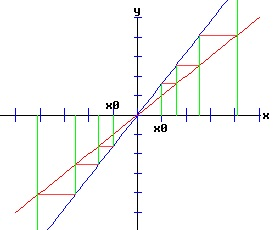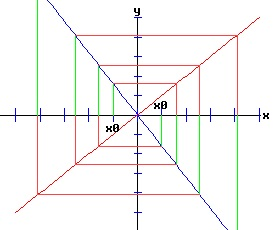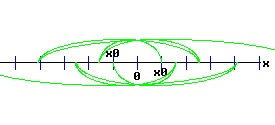The second set of diagrams above are the phase portraits of the difference equation, displaying the movement of xt along the x-axis for t = 0, 1, 2, ... etc. This sequence diverges for any initial x0 except x0 = 0. Thus, the basin of attraction of x* = 0 is the single point 0.

One Dimensional Difference Equations

The general form of a one dimensional difference equation with a parameter r is:

xt+1 = f(xt, r),   x0 = x0.         (4)

where f is a function of x and r.

Difference equations are also called maps, recursion relations, or iterated maps.

Fixed Points

For a specific value of the parameter r = r1 it is sometimes convenient to write:

f(x, r1) = f(x).         (5)

A fixed point of the function f is a number x* such that:

f(x*) = x*.         (6)

Stability Analysis

One can analyze the local stability of the difference equation (4) about a fixed point by examining the first derivative of the function f with respect to x evaluated at x* (the partial derivative of f with respect to x evaluated at x*):

λ = f'(x*) = fx(x*, r).         (7)

where λ is called the multiplier or eigenvalue, determining the stability of (4) at x*.

The discrete time, dynamic process determined by the function f is stable at x* if:

|λ| = f'(x*) < 1,

and super-stable if:

|λ| = f'(x*) = 0;

the process is unstable at x* if:

|λ| = f'(x*) > 1.

If f is stable at x*, x* is called an attractor.   If f is unstable at x*, x* is called a repeller.

If |λ| ≠ 1, x* is called a hyperbolic fixed point.

The stability of the process must be examined for each situation if:

|λ| = f'(x*) = 1.

If |λ| = 1, x* is called a non-hyperbolic fixed point.

Higher Order Maps

The second order map, f2, for the function f in (4) is given by:

xt+2  =  f(xt+1, r)  =  f (f(xt, r), r )  =   f2(xt, r)

The third order map, f3, is given by:

xt+3  = f( f (f(xt, r), r), r)  =   f3(xt, r).

Repeat this sequence for the 4th, 5th, etc. order maps.

One Dimensional Bifurcations.

A dictionary definition of bifurcation states: Division into two branches; the point of division; the branches or one of them.

A nonlinear process experiences a qualitative change in its dynamics when the nature of its fixed points change. When a change in a parameter results in a qualitative change in the dynamics of a nonlinear process, the process is said to have gone through a bifurcation.

A one dimensional, discrete time, nonlinear dynamic process is modelled by the function f of two variables, x and r, where f is nonlinear in x, and r is a parameter. The difference equation governing the dynamics of the process is:

xt+1 = f(xt, r),   x0 = x0.         (8)

Bifurcations are classified by the way in which the fixed points of the function f change their number, location, form, and stability. It is convenient to consider normal forms for the different types of bifurcations, and the conditions under which the dynamic process described by equation (8) will experience a specific type of bifurcation.

Discrete time dynamical systems may experience saddle-node, transcritical, and pitchfork bifurcations that are similar to their continuous time counterparts. The flip bifurcation is unique to discrete time dynamical processes.

A bifurcation can only occur at a non-hyperbolic fixed point.

The normal form of a discrete time process with a saddle-node bifurcation with parameter r is:

f(x, r) = a * r + b * x2,   a ≠ 0,   b ≠ 0.         (9)

Fixed points of equation (9) satisfy:

f(x, r) = a * r + b * x2 = x.         (10)

Fixed points are the roots of the quadratic equation:

b * x2 - x + a * r = 0.           (11)

For a specific value of the parameter r, the roots of equation (11) are:

x1 = (1 + sqrt(1 - 4 * b * a * r)) / (2 * b) ,         (12)

x2 = (1 - sqrt(1 - 4 * b * a * r)) / (2 * b).         (13)

Linearized Stability:

The stability of f at a fixed point, xi, is determined by the partial derivative of f with respect to x evaluated at the fixed point (ie the multiplier or eigenvalue of f):

λi = fx(xi, r) = 2 * b * xi.         (14)

Substituting (12) and (13) into (14) yields:

λ1 = 1 + sqrt(1 - 4 * b * a * r),

λ2 = 1 - sqrt(1 - 4 * b * a * r)

If the parameter r takes on the critical value:

rc = 1 / (4 * b * a),

the repeated fixed point is:

x1,2 = 1 / (2 * b),

with multiplier:

λ1,2 = 1 .

Consequently, ( x1,2, rc) = (1 / (2 * b),   1 / (4 * b * a) ) is a non-hyperbolic fixed point. In fact, it is a saddle-node bifurcation point.

Furthermore,

λ2 = 1 - sqrt(1 - 4 * b * a * r)  =  -1,   if

rc = -3 / (4 * b * a).

The fixed points are:

x1 = (1 + sqrt(1 + 3)) / (2 * b) = 3 / (2 * b),

x2 = (1 - sqrt(1 + 3)) / (2 * b) = -1 / (2 * b).

Consequently, ( x2, rc) = (-1 / (2 * b),   -3 / (4 * b * a) ) is also a non-hyperbolic fixed point. In fact, it is a flip bifurcation point.

Saddle-Node Bifurcation: a = 1, b = -1;   ( x1,2,  rc) = (- 1 / 2,  - 1 / 4 )

The prototype function f is:

f(x, r) = r - x2,         (15)

whose fixed points satisfy:

r - x2  =  x.

The fixed points and multipliers are:

x1 = (-1 - sqrt(1 + 4 * r)) / 2,   λ1 = 1 + sqrt(1 + 4 * r)

x2 = (-1 + sqrt(1 + 4 * r)) / 2,   λ2 = 1 - sqrt(1 + 4 * r)

The values of x1 and x2 are complex when r < -1 / 4, and the dynamic process has no fixed points here.

When r = -1 / 4, x1 = x2 = -1 / 2, and λ1 = λ2 = 1. The basin of attraction of the fixed point -1 / 2 is the closed interval [x1, -x1] = [-1/2,  1/2], since trajectories starting at x0 in this interval converge to -1 / 2.

When -1 / 4 < r < 3 / 4, λ1 > 1 and -1 < λ2 < 1. Consequently, x1is a repeller (unstable), and x2 is an attractor (stable). The basin of attraction for the attractor x2 is [x1, -x1].

The following diagrams display the phase diagrams and solution trajectories of x(t,  x0) for the discrete time, nonlinear dynamic process.

The yellow lines delimit the basin of attraction of the stable fixed point given by the closed interval [x1, -x1].

xt+1 = r - xt2,   x0 = x0.

 r = - .4 < -1 / 4         no real fixed points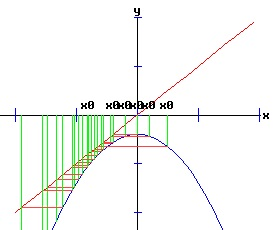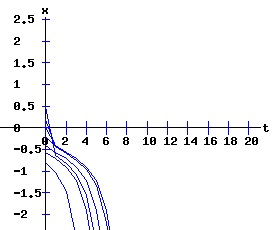r = - 1 / 4         saddle-node bifurcation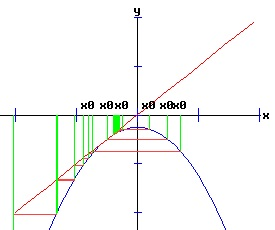r = 0         unstable & stable fixed points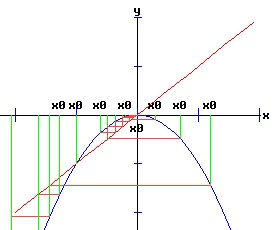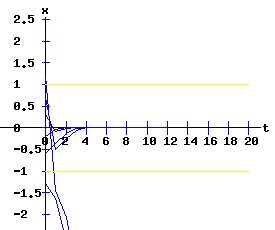r = .5         unstable & stable fixed points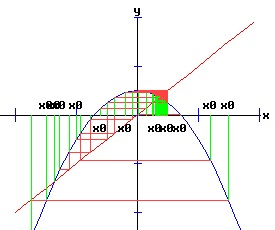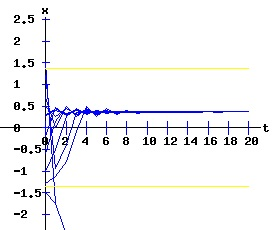Flip Bifurcation: a = 1, b = -1;   ( x2,  rc) = ( 1 / 2,  3 / 4 )

As r increases past 3 /4, the fixed point x2 loses its stability, flipping from an attractor to a repeller. The following diagrams display the phase diagrams and solution trajectories of x(t,  x0) for the discrete time, nonlinear dynamic process. The green lines delimit the minimal trapping region. If xt0 lands in the trapping region for some value of t0, xt remains in the trapping region for t > t0. This trapping region is the closed interval [f(0, r), f(f(0, r), r)], where f2(x, r) = f(f(x, r), r) is the second order map discussed below.

xt+1 = r - xt2,   x0 = x0.

 r = 3 / 4         flip bifurcation unstable fixed points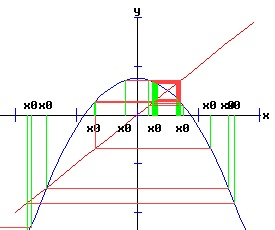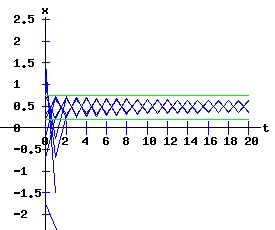r = .85         unstable fixed points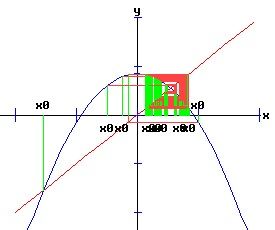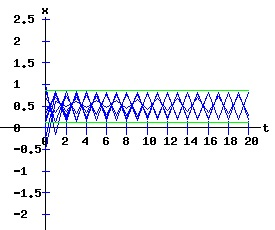r = 1         unstable fixed points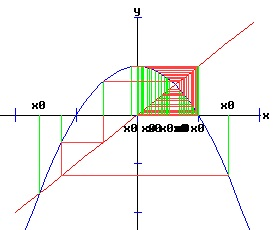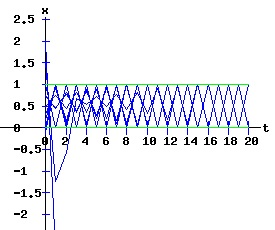r = 1.25         unstable fixed points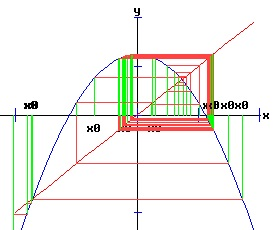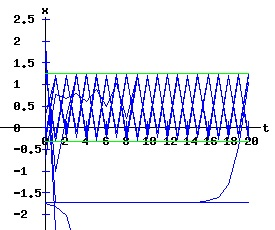r = 1.75         unstable fixed points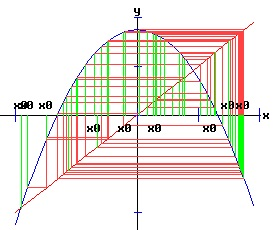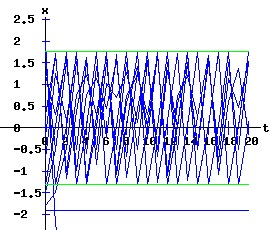The Second Order Map of f

The second order map of f as stated in (15) is the a fourth-order polynomial in x:

xt+2  =  f(xt+1, r)  =  f (f(xt, r), r ) = r - (r - xt2)2 = r - r2 + 2 * r * xt2 - xt4.         (16)

Denote this mapping by f2:

f2(x, r) = r - r2 + 2 * r * x2 - x4,         (17)

whose partial derivative with respect to x is:

fx2(x, r) = 4 * r * x - 4 * x3.         (18)

Fixed points of (17) satisfy:

f2(x, r) = r - r2 + 2 * r * x2 - x4 = x.         (19)

The fixed points of f2 are:

x1 = (-1 - sqrt(1 + 4 * r)) / 2,

x2 = (-1 + sqrt(1 + 4 * r)) / 2,

x3 = (1 + sqrt(-3 + 4 * r)) / 2,

x4 = (1 - sqrt(-3 + 4 * r)) / 2

The first two fixed points of f2are also fixed points of f.   The stability of the fixed points of f2 provide information on the bifurcation points and solution trajectories of f.

The following graphs display the phase diagrams of f in blue and f2 in red.

 r = -.4 < - 1 / 4 r = - 1 / 4 r = 0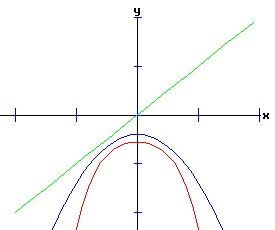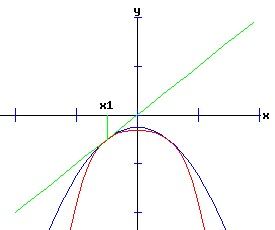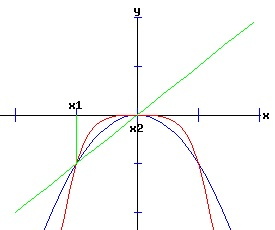r = .5 r = 3 / 4 r = .85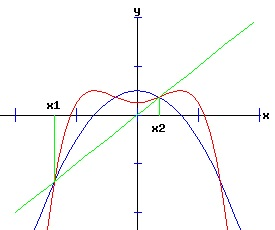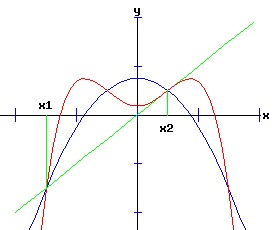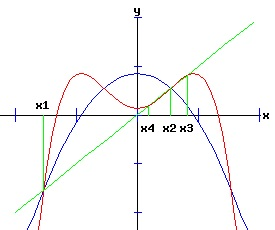r = 1 r = 1.25 r = 1.75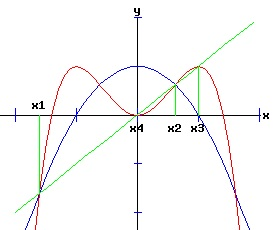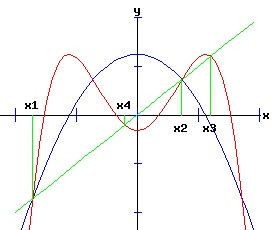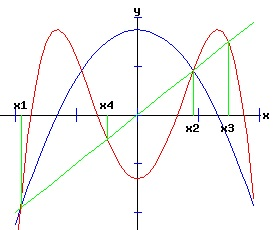At (x, r) = (-1 / 2, -1 / 4), f and f2 have a slope of 1, and f saddle-node bifurcates into two fixed points, one unstable and the other stable. At (x, r) = (1 / 2, 3 / 4), f has a slope of -1, while f2 has a slope of 1. At this flip bifurcation, the stability of f flips from stable to unstable. Meanwhile, the fixed point of f2 splits into three fixed points, bracketing the unstable fixed point that it shares with f with two stable fixed points, x3 and x4.   At r = 1.25, the fixed points x3 and x4 of f2 flip from stable to unstable.

The following bifurcation diagram summarizes the behaviour of the fixed points. It shows the fixed points as a function of the parameter r. The blue curves represent the stable fixed points, while the red curves represent the unstable fixed points. The bifurcation points are marked with an asterisk, *.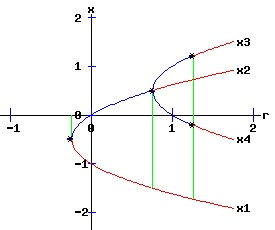This discrete time, saddle-node bifurcation diagram is similar to the continuous time, type two saddle-node bifurcation diagram.

The normal form of a discrete time process with a saddle-node bifurcation is:

xt+1 = f(xt,  r) = a * r + b * xt2,   x0 = x0,   a ≠ 0,   b ≠ 0.         (20)

The discrete time, dynamic process described by equation (20) undergoes a saddle-node bifurcation at (x*,  rc) if:

 (SN1)   f(x*, rc) - x*= 0   →   (x*, rc) is a fixed point (SN2)   fx(x*, rc) = 1   →   (x*, rc) is non-hyperbolic (SN3)   a = fr(x*, rc) ≠ 0 (SN4)   b = (1/2) * fx,x(x*, rc) ≠ 0 (21)

The bifurcation diagrams for the four types of saddle-node bifurcations are displayed below. The fixed points of f are x1 and x2, while the fixed points of f2 are x1, x2, x3, and x4. These fixed points are graphed as functions of the parameter, r. The discrete time, dynamic process undergoes a flip bifurcation when the stability of the fixed point, x2, flips. At this critical value of r, the stable fixed points x3 and x4 of f2 emerge and bracket x2.

The blue curves represent the stable fixed points, while the red curves represent the unstable fixed points. The bifurcation points are marked with an asterisk, *.

 a > 0   ,   b > 0 a > 0   ,   b < 0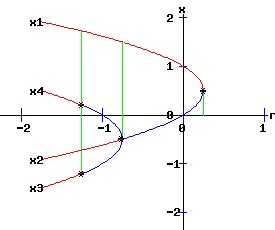a < 0   ,   b > 0 a < 0   ,   b < 0Flip Bifurcation Conditions (Lorenz 111)

Consider the discrete time, dynamic process given by:

xt+1 = f(xt,  r),   x0 = x0.         (22)

If the fixed point x = x* and parameter value r = rc satisfy:

 (F1)   f(x*, rc) - x*= 0   →   (x*, rc) is a fixed point, (F2)   fx(x*, rc) = -1   →   (x*, rc) is non-hyperbolic, (F3)   fr * fx,x + 2 * fx,r ≠ 0,   at (x*, rc), (F4) c =   -2 * fx,x,x - 3 * (fx,x)2 ≠ 0,   at (x*, rc); (23)

then, depending on the signs of the expressions in (F3) and F4),

1. the fixed point x* is stable (unstable) for r < rc (r > rc), and

2. the fixed point x* becomes unstable (stable) for r > rc (r < rc); moreover, a branch of stable (unstable) fixed points of order 2 (ie fixed points of f2) emerges which bracket x*.

Supercritical Flip Bifurcation (Period Doubling Bifurcation)

If c < 0 in expression F4, the emerging fixed points of f2 are stable and bracket an unstable fixed of f. The trajectory xt switches between the two attracting fixed points of f2.

Subcritical Flip Bifurcation

If c > 0 in expression F4, the emerging fixed points of f2 are unstable and bracket a stable fixed of f.    See the supercritical pitchfork example for an example of a subcritical flip bifurcation.

Supercritical (Period Doubling) Flip Bifurcation Example

The discrete time, dynamic process:

xt+1 = f(xt, r) = r - xt2,   x0 = x0.

with:

 fx(x, r) = -2 * x fx,x(x, r) = -2 fx,x,x(x,r) = 0 fr(x, r) = 1 fx,r(x,r) =0

undergoes a flip bifurcation at (x2, rc) = (1 / 2, 3 / 4) as r increases, where the stability of the fixed point x2 of f flips from stable to unstable, and two stable fixed points x3 and x4 of f2 emerge bracketing x2.

Checking the flip bifurcation conditions:

 (F1)  f(x2, rc) - x2 = 3/4 - (1 / 2)2 - 1 / 2 = 0, (F2)  fx(x2, rc) = -2 * 1 / 2 = -1, (F3)  fr * fx,x + 2 * fx,r = 1 * (-2) + 2 * 0 = -2 ≠ 0, (F4)  c =   -2 * fx,x,x - 3 * (fx,x)2 = -2 * 0 - 3 * (-2)2 = - 12 ≠ 0.

The supercritical flip bifurcation conditions are satisfied with c < 0.

Transcritical Bifurcation

The normal form of a discrete time process with a transcritical bifurcation with parameter r is:

xt+1 = f(xt,  r) = a * r * xt + b * xt2,   x0 = x0,   a ≠ 0,   b ≠ 0.         (24)

The fixed points of f satisfy:

f(x, r) = a * r * x + b * x2 = x.         (25)

For a specific value of the parameter r, the roots of equation (25) are:

x1 = 0 ,         (26)

x2 = (1 - a * r) / b.         (27)

Linearized Stability:

The stability of f at a fixed point, xi, is determined by the partial derivative of f with respect to x

fx(x, r) = a * r + 2 * b * x,         (28)

evaluated at the fixed point (ie the multiplier or eigenvalue of f):

λi = fx(xi, r) = a * r + 2 * b * xi.         (29)

Substituting (26) and (27) into (29) yields:

λ1 = a * r,

λ2 = - a * r + 2

If the parameter r takes on the critical value:

rc = 1 / a,

the repeated fixed point is:

x1,2 = 0,

with multiplier:

λ1,2 = 1 .

Consequently, ( x1,2, rc) = (0,   1 / a) is a non-hyperbolic fixed point. In fact, it is a transcritical bifurcation.

Furthermore,

λ1 = a * r   =   -1,   if

rc = -1 / a.

The fixed points are:

x1 = 0,

x2 = 2 / b.

Consequently, ( x1, rc) = (0,   -1 / a) is also a non-hyperbolic fixed point. In fact, it is a flip bifurcation.

Moreover,

λ2 = - a * r + 2   =   -1,   if

rc = 3 / a.

The fixed points are:

x1 = 0,

x2 = -2 / b.

Consequently, ( x2, rc) = (-2 / b,   3 / a) is also a non-hyperbolic fixed point. In fact, it is a flip bifurcation.

Transcritical Bifurcation: a = 1, b = -1;   ( x1,2,  rc) = (0,   1 )

The prototype function f is:

f(x, r) = r * x - x2,         (30)

whose fixed points satisfy:

r * x - x2  =  x.

The fixed points and multipliers are:

x1 = 0,   λ1 = r

x2 = -(1 - r),   λ2 = - r + 2

The values of x1 and x2 are real for all r.

For -1 <= r <= 1, x1 is an attractor, while x2 is a repeller. Conversely, for 1 < r <= 3, x1 is a repeller, while x2 is an attractor.

When r < 1, x1 and x2 are repellers (unstable fixed points). Similarly, when r > 3, x1 and x2 are repellers.

The following diagrams display the phase diagrams and solution trajectories of x(t,  x0) for the discrete time, nonlinear dynamic process:

xt+1 = r * xt - xt2,   x0 = x0.

The yellow linesdelimit the basin of attraction of the stable fixed point. The green lines delimit the minimal trapping region.

 r = - 2         unstable fixed points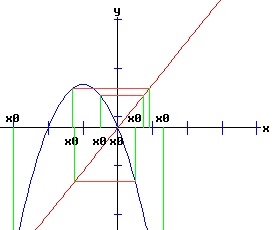r = - 1         x1 flip bifurcation point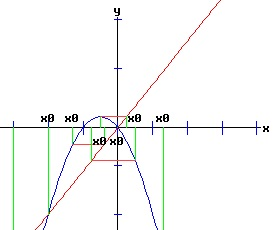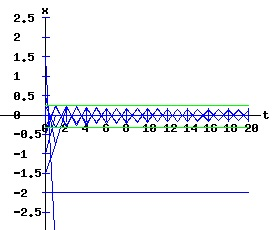r = 0         unstable & stable fixed points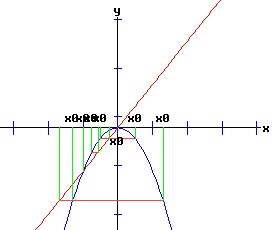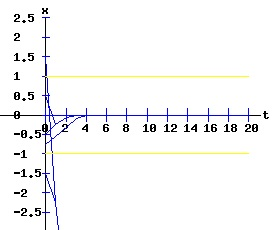r = 1         transcritical bifurcation point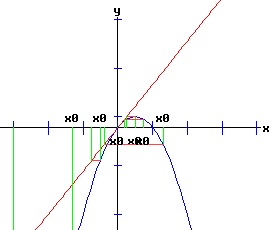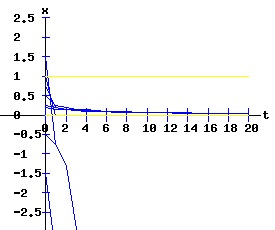r = 2         unstable and stable fixed points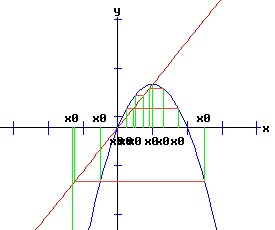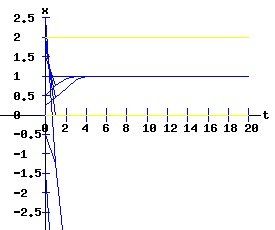r = 3         x2 flip bifurcation point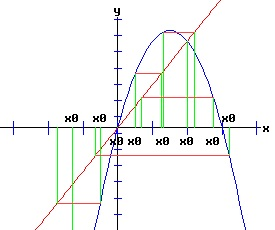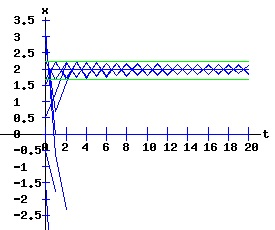r = 4         unstable fixed points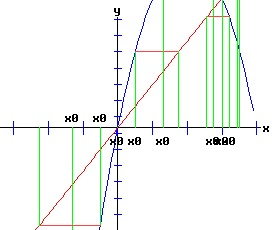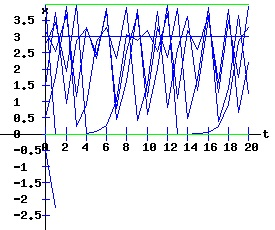The Second Order Map of f

The second order map of f as stated in (30) is the a fourth-order polynomial in x:

xt+2  =  f(xt+1, r)  =  f (f(xt, r), r ) = r * (r - xt2) - (r - xt2)2 = -xt4 + 2 * r * xt3 + (-r-r2) * xt2 + r2 * xt.         (31)

Denote this mapping by f2:

f2(x, r) = r2 * x + (-r - r2) * x2 + 2 * r * x3 - x4,         (32)

whose partial derivative with respect to x is:

fx2(x, r) = r2 + 2 * (-r - r2) * x + 6 * r * x2 - 4 * x3.         (33)

Fixed points of (32) satisfy:

f2(x, r) = r2 * x + (-r - r2) * x2 + 2 * r * x3 - x4 = x.         (34)

The fixed points of f2 are:

 x1 = 0, x2 = r - 1, x3 = (1 + r + sqrt(-3 - 2 * r + r2)) / 2, x4 = (1 + r - sqrt(-3 - 2 * r + r2)) / 2

The first two fixed points of f2are also fixed points of f.   The stability of the fixed points of f2 provide information on the bifurcation points and solution trajectories of f.

The following graphs display the phase diagrams of f in blue and f2 in red.

 r = -2 r = - 1 r = 0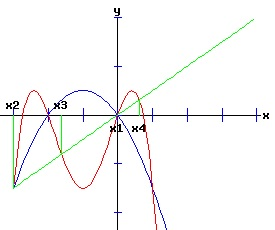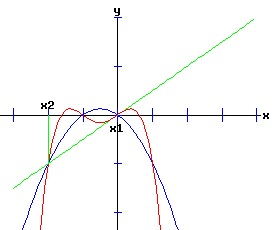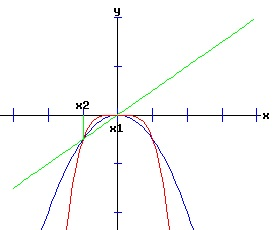r = 1 r = 3 r = 4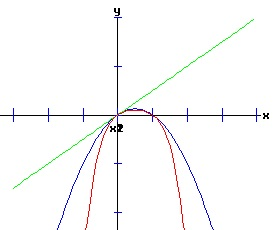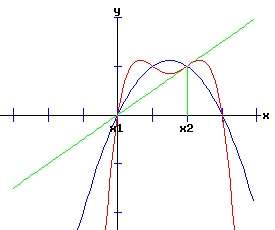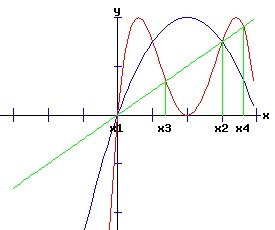At (x, r) = (0,  1), f and f2 have slopes of 1 and 1, respectively. Here f transcritical bifurcates into two fixed points x1 and x2. For -1 < r < 1, x1 is stable and x2 is unstable. At (x, r) = (0, -1), f and f2 have slopes of -1 and 1, respectively. At this flip bifurcation, the stability of x1 flips from stable to unstable for r < -1. Meanwhile, the fixed point x1 of f2 splits into three fixed points, bracketing the unstable fixed point x1 it shares with f with two stable fixed points, x3 and x4.   For 1 < r < 3, x1 is unstable and x2 is stable. At (x, r) = (x2, 3), f and f2 have slopes of -1 and 1, respectively. At this flip bifurcation, the stability of x2 flips from stable to unstable for r > 3. Meanwhile, the fixed point x2 of f2 splits into three fixed points, bracketing the unstable fixed point x2 it shares with f with two stable fixed points, x3 and x4.

The normal form of a discrete time process with a transcritical bifurcation is:

xt+1 = f(xt,  r) = a * r * xt + b * xt2,   x0 = x0,   a ≠ 0,   b ≠ 0.         (35)

Transcritical Conditions

 (TC1)   f(0, rc) = 0 for all r (TC2)   fx(0, rc) = 1   →   (0, rc) is non-hyperbolic (TC3)   a = fx,r(0, rc) ≠ 0 (TC4)   b = (1/2) * fx,x(0, rc) ≠ 0 (36)

The bifurcation diagrams for the four types of transcritical bifurcations are displayed below. The fixed points of f are x1 and x2, while the fixed points of f2 are x1, x2, x3, and x4. These fixed points are graphed as functions of the parameter, r. The discrete time, dynamic process undergoes supercritical flip bifurcations when the stability of the fixed points, x1 and x2, flip. At these critical values of r, the stable fixed points x3 and x4 of f2 emerge and bracket x1 or x2.

The blue curves represent the stable fixed points, while the red curves represent the unstable fixed points. The bifurcation points are marked with an asterisk, *.

 a > 0   ,   b > 0 a > 0   ,   b < 0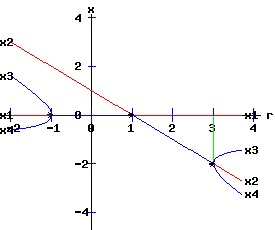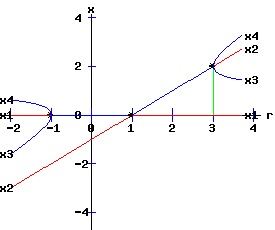a < 0   ,   b > 0 a < 0   ,   b < 0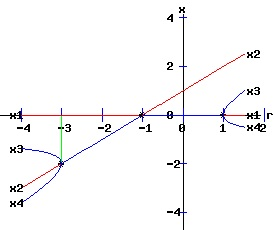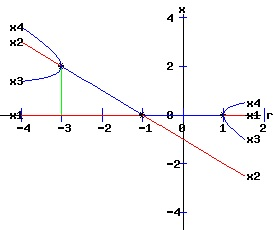PitchFork Bifurcation

The normal form of a discrete time process with a pitchfork bifurcation with parameter r is:

xt+1 = f(xt,  r) = a * r * xt + b * xt3,   x0 = x0,   a ≠ 0,   b ≠ 0.         (37)

Supercritical Pitchfork Bifurcation

In the normal form of a supercritical pitchfork bifurcation, the coefficient, b, in equation (37) is negative. Consequently, the cubic term stabilizes the dynamic process by pulling the trajectory of x(t) back toward x = 0.

Subcritical Pitchfork Bifurcation

In the normal form of a subcritical pitchfork bifurcation, the coefficient, b, in equation (37) is positive. Consequently, the cubic term destabilizes the dynamic process by driving the trajectory of x(t) toward infinity.

The fixed points of f satisfy:

f(x, r) = a * r * x + b * x3 = x.         (38)

For a specific value of the parameter r, the roots of equation (38) are:

x1 = 0 ,         (39)

x2 = sqrt(-b * (a * r - 1)) / b.         (40)

x3 = -sqrt(-b * (a * r - 1)) / b.         (41)

The fixed points x1 and x2 are real if:

-b * (a * r - 1) > 0.         (42)

Linearized Stability:

The stability of f at a fixed point, xi, is determined by the partial derivative of f with respect to x

fx(x, r) = a * r + 3 * b * x2,         (43)

evaluated at the fixed point (ie the multiplier or eigenvalue of f):

λi = fx(xi, r) = a * r + 2 * b * xi2.         (44)

Substituting (39), (40), and (41) into (44) yields:

λ1 = a * r,

λ2 = -2 * a * r + 3

λ3 = -2 * a * r + 3

If the parameter r takes on the critical value:

rc = 1 / a,

the triple fixed point is:

x1,2,3 = 0,

with multiplier:

λ1,2,3 = 1 .

Consequently, ( x1,2,3, rc) = (0,   1 / a) is a non-hyperbolic fixed point. In fact, it is a pitchfork bifurcation.

Furthermore,

λ1 = a * r   =   -1,   if

rc = -1 / a,

with corresponding fixed point:

x1 = 0.

With r = - 1 / a, the fixed points x2 and x3 are real if b > 0, with

x2 = sqrt(2 * b) / b,

x3 = -sqrt(2 * b) / b.

Moreover,

λ2,3 = - 2 * a * r + 3   =   -1,   if

rc = 2 / a.

With r = 2 / a, the fixed points x2 and x3 are real if b < 0, with

x1 = 0,

x2 = sqrt(- b) / b,

x3 = -sqrt(- b) / b.

Supercritical PitchFork Bifurcation: a = 1, b = -1;   ( x1,2,3,  rc) = (0,   1 )

The prototype function f is:

f(x, r) = r * x - x3,         (45)

whose fixed points satisfy:

r * x - x3  =  x.

The fixed points and multipliers are:

x1 = 0,   λ1 = r

x2 = -sqrt(r - 1),   λ2 = - 2 * r + 3

x3 = sqrt(r - 1),   λ2 = - 2 * r + 3

The values of x2 and x3 are real for all r >= 1.

For r < -1, x1 is a repeller. At r = -1, x1 flips to attractor. For -1 < r < 1, x1 is an attractor. At r = 1, x1 flips to repeller. For 1 < r <= 2, x2 and x3 are attractors. At r = 2, x2 and x3 flip to repellers for r > 2.

The following diagrams display the phase diagrams and solution trajectories of x(t,  x0) for the discrete time, nonlinear dynamic process:

xt+1 = r * xt - xt3,   x0 = x0.

 r = - 2         unstable fixed points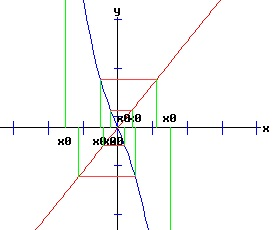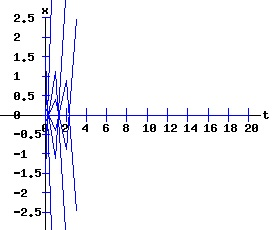r = - 1         x1 flip bifurcation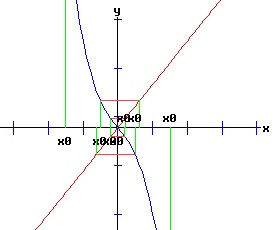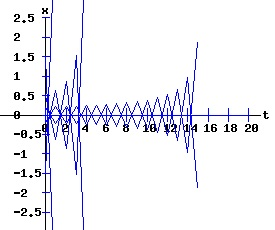r = 0         x1 stable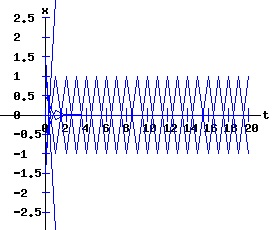r = 1         x1 pitchfork bifurcation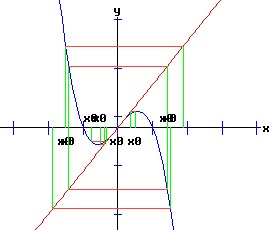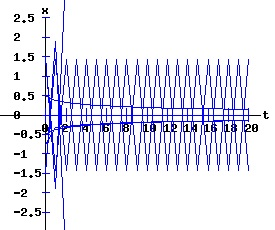r = 1.5         stable and unstable and stable fixed points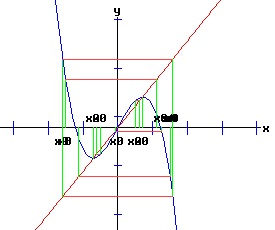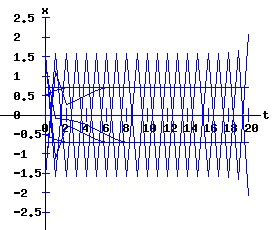r = 2         x2 and x3 flip from stable to unstable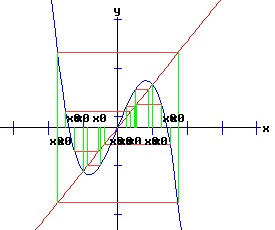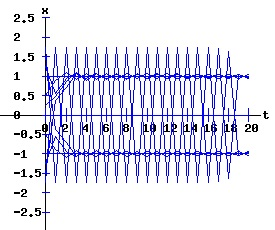r = 3         unstable fixed points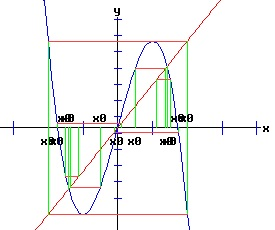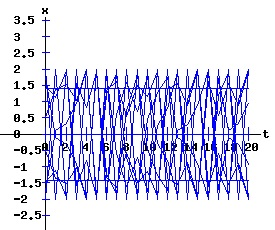The Second Order Map of f

The second order map of f as stated in (45) is the a ninth-order polynomial in x:

xt+2  =  f(xt+1, r)  =  f (f(xt, r), r ) = r * (r - xt3) - (r - xt3)3 = xt9 - 3 * r * xt7 + 3 * r2 * xt5 + (-r - r3) * xt3 + r2 * xt.         (46)

Denote this mapping by f2:

f2(x, r) = r2 * x + (-r - r3) * x3 + 3 * r2 * x5 - 3 * r * x7 + x9,         (47)

whose partial derivative with respect to x is:

fx2(x, r) = r2 + 3 * (-r - r3) * x2 + 15 * r2 * x4 - 21 * r * x6 + 9*x8.         (48)

Fixed points of (47) satisfy:

f2(x, r) = r2 * x + (-r - r3) * x3 + 3 * r2 * x5 - 3 * r * x7 + x9 = x.         (49)

These fixed points of f2 are:

 x1 = 0, x2 = -sqrt(r - 1), x3 = sqrt(r - 1), x4 = -sqrt(r + 1,) x5 = sqrt(r + 1), x6 = -sqrt(2 * r - 2 * sqrt(r2 - 4)) / 2, x7 = sqrt(2 * r - 2 * sqrt(r2 - 4)) / 2, x8 = -sqrt(2 * r + 2 * sqrt(r2 - 4)) / 2, x9 = sqrt(2 * r + 2 * sqrt(r2 - 4)) / 2.

The first three fixed points of f2are also fixed points of f. When r >= 1, x2 and x3 are real numbers. When r >= -1, x4 and x5 are real numbers. When r >= 2, x6, x7, x8, and x9 emerge as real numbers.

The following graphs display the phase diagrams of f in blue and f2 in red.

 r = -1 r = 0 r = 1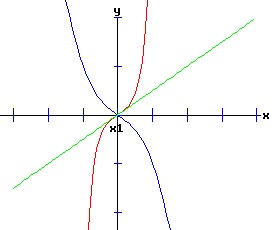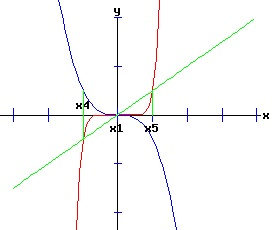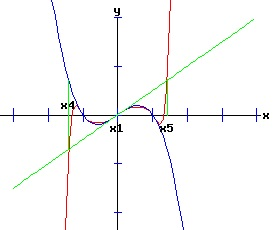r = 1.5 r = 2 r = 3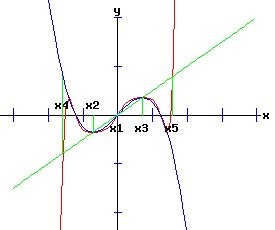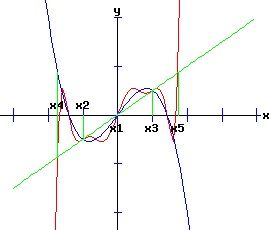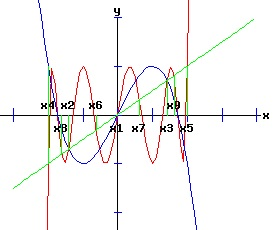At (x, r) = (0,  1), f and f2 each have a slope of 1; f pitchfork bifurcates with two new fixed points x2 and x3 emerging as r increases. At (x, r) = (0, -1), f and f2 have slopes of -1 and 1, respectively. At this flip bifurcation, the stability of x1 flips from unstable to stable as r increases. Meanwhile, the fixed point x1 of f2 splits into three fixed points, bracketing the stable fixed point x1 it shares with f with two unstable fixed points, x4 and x5. For -1 < r < 1, x1 is stable and x4 and x5 are unstable.   For 1 < r < 2, x1 is unstable, while x2 and x3 are stable. At r = 2, f and f2evaluated at x2 and x3 have slopes of -1 and 1, respectively. At this flip bifurcation, the stabilities of x2 and x3 flip from stable to unstable for r > 3. Meanwhile, the fixed points x2 and x3 of f2 each split into three fixed points, bracketing the unstable fixed point shared with f with stable fixed points, x6 and x8 around x2, x7 and x9 around x3.

The normal form of a discrete time process with a pitchfork bifurcation is:

xt+1 = f(xt,  r) = a * r * xt + b * xt3,   x0 = x0,   a ≠ 0,   b ≠ 0.         (35)

Note that f is an odd function since:

f(-x, r) = -f(x, r),

and therefore function f does not have a transcritical bifurcation since:

fx,x(0, r) = 0.

Pitchfork Conditions

 (PF1)   f(-x, r) = -f(x, r)   →   f is an odd function   →   f(0, r) = 0 (PF2)   fx(0, rc) = 1   →   (0, rc) is non-hyperbolic (PF3)   a = fx,r(0, rc) ≠ 0 (PF4)   b = (1/6)* fx,x,x(0, rc) ≠ 0 (36)

Bifurcation Diagrams

The bifurcation diagrams for the two types of supercritical and two types of subcritical pitchfork bifurcations are displayed below. The fixed points of f are x1, x2, and x3, whereas the fixed points of f2 are x1, x2, x3, x4, x5, x6, x7, x8, and x9. These fixed points are graphed as functions of the parameter, r.

Supercritical Pitchfork Bifurcation Diagrams (b < 0)

The discrete time, dynamic process undergoes a pitchfork bifurcation at r = 1 / a where x1 bifurcates into x1, x2, and x3. A subcritical flip bifurcation occurs at r = -1 / a where the stability of the fixed point x1 flips, and the unstable fixed points x4 and x5 of f2 emerge bracketing the stable fixed point x1 of f. Supercritical flip bifurcations occur at r = 2 /a where the stabilities of x2 and x3 flip from stable to unstable. Concurrently, the stable fixed points x6 and x8 of f2 emerge bracketing x2, and the stable fixed points x7 and x9 of f2 emerge bracketing x3.

The blue curves represent the stable fixed points, whereas the red curves represent the unstable fixed points. A bifurcation point is marked with an asterisk, *.

 a > 0   ,   b < 0 a < 0   ,   b < 0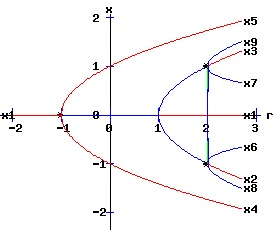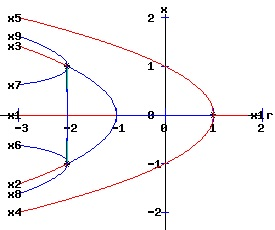Subcritical Pitchfork Bifurcation Diagrams (b > 0)

The discrete time, dynamic process undergoes a pitchfork bifurcation at r = 1 / a where x1 bifurcates into x1, x2, and x3. A supercritical flip bifurcation occurs at the critical value of r = -1 / a where the stability of the fixed point x1 flips. Concurrently, fixed points of f2 emerge bracketing x1 with x4 and x5. At r = -2 / a, the fixed point x4 of f2 bifurcates into x4, x6 and x8; meanwhile the fixed point x5 of f2 bifurcates into x5, x7 and x9.

The blue curves represent the stable fixed points, whereas the red curves represent the unstable fixed points. A bifurcation point is marked with an asterisk, *.

a > 0   ,   b > 0

a < 0   ,   b > 0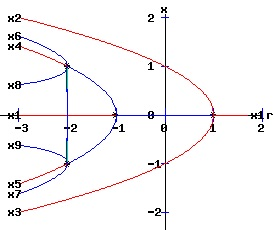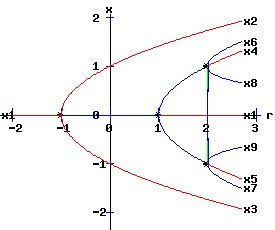References.

• Burden, Richard L. and J. Douglas Faires. Numerical Analysis. 6th ed. Pacific Grove: Brooks/Cole, 1997.
• Demmel, James W. Applied Numerical Linear Algebra. Philadelphia: Siam, 1997.
• Devaney, Robert L. An Introduction to Chaotic Dynamical Systems. Menlo Park, CA: Benjamin/Cummings, 1986.
• Intriligator, M. D. Mathematical Optimization and Economic Theory. Englewood Cliffs: Prentice-Hall, 1971.Copyright © Elmer G. Wiens:   Egwald Web ServicesAll Rights Reserved.    Inquiries